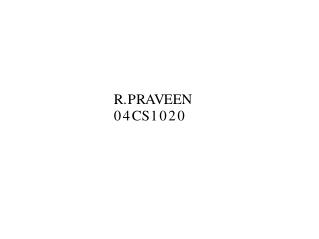DownloadDownload PresentationLL PARSER

# LL PARSER

Download Presentation## LL PARSER

- - - - - - - - - - - - - - - - - - - - - - - - - - - E N D - - - - - - - - - - - - - - - - - - - - - - - - - - -
##### Presentation Transcript

1. The construction of a predictive parser is aided by two functions associated with a grammar called FIRST and FOLLOW. These functions allows us to fill the entries of the predictive parsing table. LL PARSER

2. We associate each grammar symbol A with the set FIRST(A). The implication of this set is that the grammar symbol A can in some steps of transition produce the elements of the set FIRST(A). If 'α' is any string of grammar symbols, let FIRST(α) be the set of terminals that begin the string derived from α . If α=*=>є then add є to FIRST(є). FIRST

3. 1) If X is a terminal , then FIRST(X) is {X} 2) If X--> є then add є to FIRST(X) 3) If X is a nonterminal and X-->Y1Y2Y3...Yn , then put 'a' in FIRST(X) if for some i, a is in FIRST(Yi) and є is in all of FIRST(Y1),...FIRST(Yi-1); In other words, Y1...Yi-1=*=>є . If є is in all of FIRST(Yj) for all j=1,2....n, then add є to FIRST(X).This is so because all Yi's produce є, so X definitely produces є RULES TO COMPUTE FIRST SET

4. FOLLOW is defined only for non terminals of the grammar G. It can be defined as the set of terminals of grammar G , which can immediately follow the non terminal in a production rule from start symbol. In other words, if A is a nonterminal, then FOLLOW(A) is the set of terminals 'a' that can appear immediately to the right of A in some sentential form, i.e. The set of terminals 'a' such that there exists a derivation of the form S=*=> αAaβ for some αandβ (which can be strings ,or empty). FOLLOW

5. 1)If S is the start symbol, then add \$ to the FOLLOW(S). 2)If there is a production rule A--> αBβ then everything in FIRST(β) except for є is placed in FOLLOW(B). 3)If there is a production A--> αB , or a production A--> αBβ where FIRST(β) contains єthen everything in FOLLOW(A) is in FOLLOW(B). RULES TO COMPUTE FOLLOW SET

6. Consider the following grammar S-> aABe A-->Abc | b B--> d Find the FIRST and FOLLOW for each nonterminal of the grammar. Example

7. Steps : Find for every non terminal if it is nullable. Find FIRST for every nonterminal using rules described earlier. Using the information got from calculations in steps 1 and 2 one could calculate FOLLOW for every nonterminal by rules described earlier. Solution

8. a) To calculate FIRST(S) : From rule S-->aABe , we get 'a' belongs to FIRST(S) No other rule will help give an element in FIRST(S). So, FIRST(S)={a} b) To calculate FIRST(A) : From rule A-->Abc , we can't add any element From rule A-->b , we know that 'b' belongs to FIRST(A) No other rule will help give an element in FIRST(A). So, FIRST(A)={b} c) To calculate FIRST(B) From rule B-->d , we add 'd' to FIRST(B) No other rule will help give an element in FIRST(B). So, FIRST(B)={d} To calculate FIRST’s

9. a) To calculate FOLLOW(S) Since S is start symbol, add \$ to FOLLOW(S) From rule S-->aABe , we don’t get any contribution to the FOLLOW(S) /*See rules 2 and 3 for FOLLOW*/ From rule A-->Abc , since no where we see any symbol S, so no contribution to FOLLOW(A) is found in this rule. From rule A-->b , again no contribution. From rule B-->d, again no contribution. Hence FOLLOW(S) ={\$} To calculate FOLLOW’s

10. b) To calculate FOLLOW(A) : From rules A-->b , B-->d we get no contribution. From rule S-->aABe we expect to get some contribution. See rule 2 of forming FOLLOW(on page 2) As per that , we can add everything in FIRST(Be) in FOLLOW(A) except for epsilon. FIRST(Be)=FIRST(B) ={d} So add 'd' to FOLLOW(A). Since Be is not nullable, so we can't use the rule 3 (See page 2) For rule A-->Abc , we do get some contribution straight away. From rule 2(page 2), 'b' belongs to FOLLOW(A) No other rule will help give an element in FOLLOW(A). Hence FOLLOW(A)={d,b}

11. c) To calculate FOLLOW(B) Only rule S-->aABe contributes. By rule 2 , 'e' belongs to FOLLOW(B). Hence FOLLOW(B)={e}